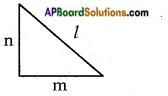## AP State Syllabus SSC 10th Class Maths Solutions 8th Lesson Similar Triangles InText Questions

AP State Board Syllabus AP SSC 10th Class Maths Textbook Solutions Chapter 8 Similar Triangles InText Questions and Answers.

### 10th Class Maths 8th Lesson Similar Triangles InText Questions and Answers

Do these

(Page No. 194)

Question 1.
Fill in the blanks with similar/not similar.
i) All squares are ………. (similar)
ii) All equilateral triangles are ………. (similar)
iii) All isosceles triangles are ………. (similar)
iv) Two polygons with same number of sides are ………, if their corresponding angles are equal and corresponding sides are equal. (similar)
v) Reduced and enlarged photographs of an object are ………. (similar)
vi) Rhombus and squares are ……… to each other. (not similar)Question 2.
Write True / False for the following statements.
i) Any two similar figures are congruent.
False
ii) Any two congruent figures are similar.
True
iii) Two polygons are similar if their corresponding angles are equal.
False

Question 3.
Give two different examples of pair of
i) Similar figures
ii) Non-similar figures
i) Similar figures:
a) Any two circles
b) Any two squares
c) Any two equilateral triangles
ii) Non-similar figures:
a) A square and a rhombus
b) A square and a rectangle

Question 4.
What value(s) of x will make DE || AB, in the given figure?  (Page No. 200)
AD = 8x + 9, CD = x + 3,
BE = 3x + 4, CE = x.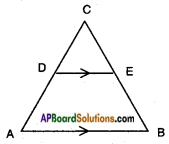Given : In AABC, DE // AB AD = 8x + 9, CD = x + 3,
BE = 3x + 4, CE = x
By Basic proportionality theorem,
If DE // AB then we should have
$$\frac{\mathrm{CD}}{\mathrm{DA}}$$ = $$\frac{\mathrm{CE}}{\mathrm{EB}}$$
$$\frac{x+3}{8x+9}$$ = $$\frac{x}{3x+4}$$
⇒ (x + 3) (3x + 4) = x (8x + 9)
⇒ x (3x + 4) + 3 (3x + 4) – 8x2 + 9x
⇒ 3x2 + 4x + 9x + 12 = 8x2 + 9x
⇒ 8x2 + 9x – 3x2 – 4x – 9x -12 = 0
⇒ 5x2 – 4x – 12 = 0
⇒ 5x2 – 10x + 6x – 12 = 0
⇒ 5x (x – 2) + 6 (x – 2) = 0
⇒ (5x + 6) (x – 2) = 0
⇒ 5x + 6 = 0 or x – 2 = 0
⇒ x = $$\frac{-6}{5}$$ or x = 2;
x cannot be negative.
∴ The value x = 2 will make DE // AB.Question 5.
In △ABC, DE || BC. AD = x, DB = x – 2, AE = x + 2 and EC = x – 1. Find the value of x.    (Page No. 200)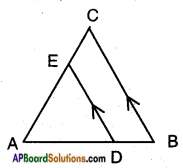Given: In △ABC, DE // BC
∴ By Basic proportionality theorem, we have
$$\frac{\mathrm{AD}}{\mathrm{DB}}$$ = $$\frac{\mathrm{AE}}{\mathrm{EC}}$$
$$\frac{\mathrm{x}}{\mathrm{x}-2}$$ = $$\frac{x+2}{x-1}$$
⇒ x (x – 1) = (x + 2) (x – 2)
⇒ x2 – x = x2 – 4
⇒ -x = -4
∴ x = 4

Try This

Question 1.
E and F are points on the sides PQ and PR respectively of △PQR. For each of the following, state whether EF || QR or not?      (Page No. 197)
i) PE = 3.9 cm, EQ = 3 cm,
PF = 3.6 cm and FR = 2.4 cm.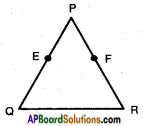Here
$$\frac{\mathrm{PE}}{\mathrm{EQ}}$$ = $$\frac{3.9}{3}$$ = $$\frac{1.3}{1}$$
$$\frac{\mathrm{PF}}{\mathrm{FR}}$$ = $$\frac{3.6}{2.4}$$ = $$\frac{3}{2}$$
$$\frac{\mathrm{PE}}{\mathrm{EQ}}$$ ≠ $$\frac{\mathrm{PF}}{\mathrm{FR}}$$
Hence, EF is not parallel to QR.ii) PE = 4 cm, QE = 4.5 cm,
Here
$$\frac{\mathrm{PE}}{\mathrm{EQ}}$$ = $$\frac{4}{4.5}$$ = $$\frac{8}{9}$$
$$\frac{\mathrm{PF}}{\mathrm{RF}}$$ = $$\frac{8}{9}$$
$$\frac{\mathrm{PE}}{\mathrm{EQ}}$$ = $$\frac{\mathrm{PF}}{\mathrm{RF}}$$
∴ EF // QR
Hence, EF is parallel to QR.

iii) PQ = 1.28 cm, PR = 2.56 cm, PE = 1.8 cm and PF = 3.6 cm.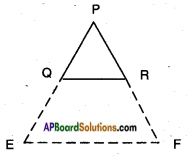Given: PQ = 1.28 cm, PE = 1.8 cm
⇒ EQ = PE – PQ = 1.8 – 1.28
⇒ EQ = 0.52 cm
Also, PR = 2.56 cm, PE = 3.6 cm, FR = PF – PR = 3.6 cm – 2.56 cm
FR = 1.04 cm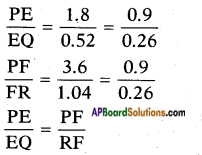∴ EF // QR (By converse of Basic proportionality theorem)
Hence, EF is parallel to QR.

Question 2.
In the following figures DE || BC.    (Page No. 198)
i) Find EC.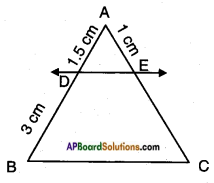$$\frac{\mathrm{AD}}{\mathrm{DB}}$$ = $$\frac{AE}{EC}$$
⇒ $$\frac{1.5}{3}$$ = $$\frac{1}{EC}$$
∴ EC = $$\frac{3}{1.5}$$ = 2 cm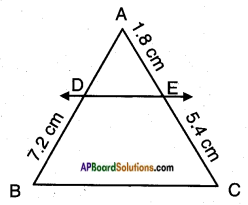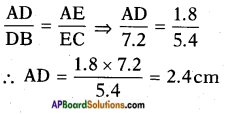Think & Discuss

Question 1.
Can you give some more examples from your daily life where scale factor is used?    (Page No. 192)
Scale factor is used in drawing maps, designing machines and in sculpture, etc.

Question 2.
Can you say that a square and a rhombus are similar? Discuss with your friends.Write why the conditions are not sufficient.       (Page No. 193)
A square □ ABCD and a rhombus ▱ PQRS are not similar.
Though the ratio of their corresponding sides are equal, the corresponding angles are not equal.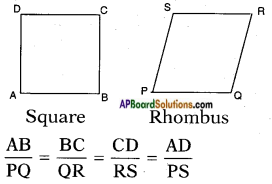but ∠A ≠ ∠P; ∠B ≠ ∠Q
∠C ≠ ∠R; ∠D ≠ ∠S

Try This

(Page No. 207)

Question 1.
Are the triangles similar ? If so, name the criterion of similarity. Write the similarity relation in symbolic form.
i)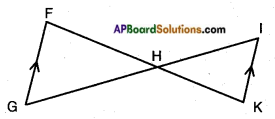!! ∠G = ∠I alt. int. angles for the ∠F = ∠K parallel lines GF // KI
∠FHG = ∠IHK (Vertically opp. angle)
∴ ∠GHF and ∠IKH are similar by AAA similarity rule.
△GHF ~ △IKH.

ii)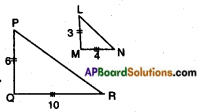$$\frac{PQ}{LM}$$ = $$\frac{6}{3}$$ = 2;
$$\frac{QR}{MN}$$ = $$\frac{10}{4}$$ = 2.5;
$$\frac{PQ}{LM}$$ ≠ $$\frac{QR}{MN}$$
△PQR and △LMN are not similar.
△PQR ~ △LMNiii)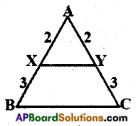∠A = ∠A (Common)
$$\frac{AB}{AX}$$ = $$\frac{5}{3}$$; $$\frac{AC}{AY}$$ = $$\frac{5}{3}$$
∴ △ABC and △AXY are similar by SAS similarity condition.
△ABC ~ △AXY.

iv)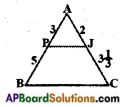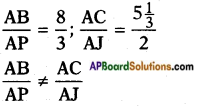∴ △ABC and △APJ are not similar.

v)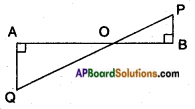∠A = ∠B = 90°
∠AOQ = ∠POB (∵ Vertically opposite angles)
∠Q = ∠P (alternate interior angles)
∴ △AOQ and △BOP are similar by AAA criterion.
△AOQ ~ △BOP.vi)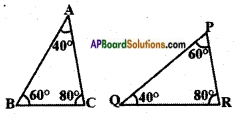△ABC and △QPR are similar by AAA similarity condition.
△ABC ~ △QPR.

vii)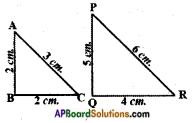∠A = ∠P
$$\frac{AB}{PQ}$$ = $$\frac{2}{5}$$; $$\frac{AC}{PR}$$ = $$\frac{3}{6}$$ = $$\frac{1}{2}$$
∴ $$\frac{AB}{PQ}$$ ≠ $$\frac{AC}{PR}$$
Hence not similar.

viii)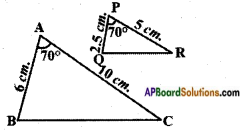$$\frac{AB}{PQ}$$ = $$\frac{6}{2.5}$$; $$\frac{AC}{PR}$$ = $$\frac{10}{5}$$
∴ △ABC and △PQR are not similar.

Question 2.
Explain why the triangles are similar and then find the value of x.
i)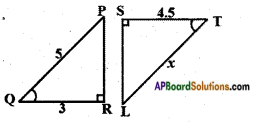Given: In △PQR and △LTS
∠Q = ∠T; ∠R = ∠S = 90°
∴ ∠P = ∠T
(by angle sum property of triangles)
Hence, △PQR ~ △LTS [∵ AAA]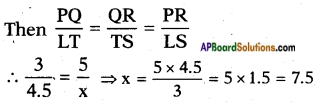ii)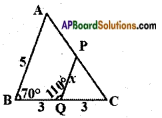Given: In △ABC and △PQC
∠B = ∠Q
[∵ ∠PQC = 180°- 110° = 70° – linear pair of angles]
∠C = ∠C [∵ Common]
∠A = ∠P [∵ Angle Sum property of triangles]
△ABC ~ △PQC by AAA similarity condition.
Then the ratio of their corresponding sides are equal.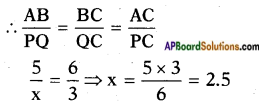iii)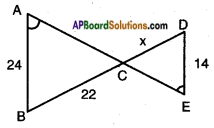Given: In △ABC and △ECD
∠A = ∠E
∠ACB = ∠ECD [∵ Vertically opposite]
∴ ∠B = ∠D [∵ angle sum property]
∴ △ABC ~ △EDC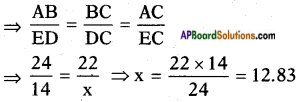iv)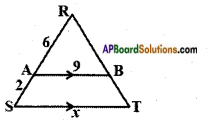Given: In △RAB and △RST
∠R = ∠R (common)
∠A = ∠S and ∠B = ∠T
[∵ Pair of corresponding angles for AB // ST]
∴ △RAB ~ △RST [∵ AAA similarity]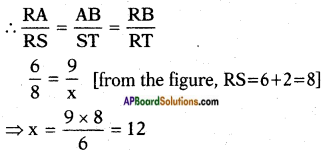v)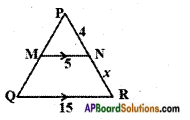Given: In △PQR and △PMN
∠P = ∠P [∵ Common]
∠Q = ∠M [∵ Pair of corresponding angles for MN // QR]
∠R = ∠N
△PQR ~ △PMN [∵ AAA similarity]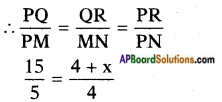[From the figure, PR = 4 + x]
⇒ 3 × 4 = 4 + x
⇒ x = 12 – 4 = 8

vi)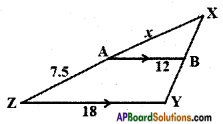Given: In △XYZ and △XBA,
∠X = ∠X [∵ Common]
∠B = ∠Y [∵ Pair of corresponding ∠A = ∠Z angles for AB // ZY]
∴ △XYZ ~ △XBA [∵ AAA similarity]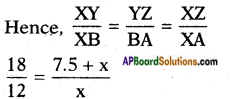[From the figure, XZ = 7.5 + x]
$$\frac{3}{2}$$ = $$\frac{7.5+\mathrm{x}}{\mathrm{x}}$$
3x = 15 + 2x;
3x – 2x = 15
x = 15

vii)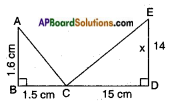Given: With the given conditions, we can’t find the value of x.
Note: If it is given that ∠A = ∠E then
we can say that △ABC ~ △EDC by AAA rule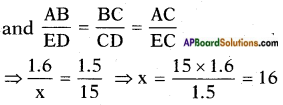viii)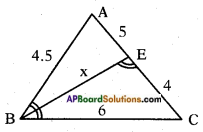In △ABC and △BEC
∠ABC = ∠CEB (given)
∠C = ∠C (Common angle)
∴ △ABC ~ △BEC
(A.A. Criterion similarity)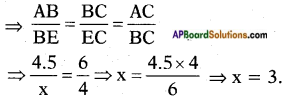Think & Discuss

(Page No. 203)

Question 1.
Discuss with your friends that in what way similarity of triangles is dif¬ferent from similarity of other polygons?
In two triangles if the corresponding angles are equal then they are similar, whereas in two polygons if the corre-sponding angles are equal, they may not be similar, i.e., In triangles,
(Pairs of corresponding angles are equal) ⇔ (Ratio of corresponding sides are equal).
But this is not so with respect to polygons.

Do ThisQuestion 1.
In △ACB, ∠C = 90° and CD ⊥ AB. Prove that $$\frac{\mathrm{BC}^{2}}{\mathrm{AC}^{2}}$$ = $$\frac{BD}{AD}$$.    (Page No. 218)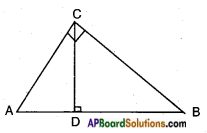Proof: △ADC and △CDB are similar.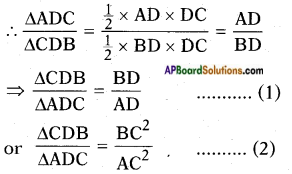[Ratio of areas of similar triangles is equal to the ratio of squares of their corresponding sides.]
From (1) and (2),
$$\frac{BD}{AD}$$ = $$\frac{\mathrm{BC}^{2}}{\mathrm{AC}^{2}}$$ (Q.E.D.)

Question 2.
A ladder 15 m long reaches a window which is 9 m above the ground on one side of a street. Keeping its foot at the same point, the ladder is turned to other side of the street to reach a window 12 m high. Find the width of the street.    (Page No. 218)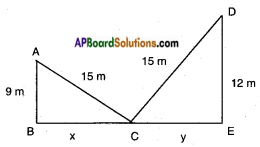Let A and D be the windows on the either sides of the street.
From Pythagoras theorem,
AC2 = AB2 + BC2
152 = 92 + BC2
BC2 = 225 – 81
BC = √144 = 12 ….. (1)
Also, CD2 = DE2 + CE2
152 = 122 + CE2
CE2 = 225 – 144
CE = √81 = 9
∴ BE = BC + CE = 12 + 9 = 21
Width of the street = 21 m.Question 3.
In the given figure if AD ⊥ BC, prove that AB2 + CD2 = BD2 + AC2.    (Page No. 219)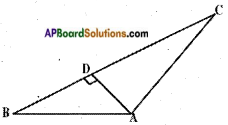Given: In △ABC, AD ⊥ BC.
R.T.P: AB2 + CD2 = BD2 + AC2
Proof: △ABD is a right angled triangle
AB2 – BD2 = AD2 ……. (1)
△ACD is a right angle triangle
From (1) and (2)
AB2 – BD2 = AC2 – CD2
AB2 + CD2 = BD2 + AC2

Think & Discuss

Question 1.
For a right angled triangle with integer sides atleast one of its measurements must be an even number. Why? Discuss this with your friends and teachers.    (Page No. 215)